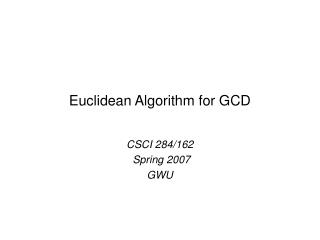DownloadDownload PresentationEuclidean Algorithm for GCD

# Euclidean Algorithm for GCD

Download Presentation## Euclidean Algorithm for GCD

- - - - - - - - - - - - - - - - - - - - - - - - - - - E N D - - - - - - - - - - - - - - - - - - - - - - - - - - -
##### Presentation Transcript

1. Euclidean Algorithm for GCD CSCI 284/162 Spring 2007 GWU

2. Euclidean Algorithmconsidered first non-trivial algorithm Algorithm_gcd(m, a) /* m > a */ (X, Y) := (m, a) /* Initialize */ while (Y0) (X, Y) := (Y, X rem Y) return(X) Works because: gcd (X, Y) = gcd(Y, X rem Y) gcd(X, Y) = Y if Y|X Stops because: (X, Y) always decreasing and non-negative CS284-162/Spring07/GWU/Vora/ Euuclidean Algorithm for GCD

3. Try gcd(17, 101) gcd(57, 93) CS284-162/Spring07/GWU/Vora/ Euuclidean Algorithm for GCD

4. Theorem: gcd(X, Y) = gcd(Y, X rem Y); X, Y0 Proof: Let X rem Y = r  r = X – qY for integer q Consider a factor g of X and Y g|X and g|Y • X = q1g, Y = q2g, for some integers q1 and q2 • r = g(q1 – qq2) • g|Y and g|r CS284-162/Spring07/GWU/Vora/ Euuclidean Algorithm for GCD

5. Theorem: gcd(X, Y) = gcd(Y, X rem Y); X, Y0 Proof contd: Consider a factor h of Y and r h|Y and h|r • Y = q3h, r = q4h for some integers q3 and q4 • X = h(q4 + qq3) • h|Y and h|X Note: all integers are factors of 0, see, for example, example 2.80 in Chapter 2, Handbook of Applied Cryptography, http://www.cacr.math.uwaterloo.ca/hac/about/chap2.pdf CS284-162/Spring07/GWU/Vora/ Euuclidean Algorithm for GCD

6. Theorem: gcd(X, Y) = gcd(Y, X rem Y)Proof contd. Hence all common factors of X and Y are common factors of Y and r and vice versa. Hence, in particular, the pair (X, Y) and the pair (Y, r) have the same gcd. CS284-162/Spring07/GWU/Vora/ Euuclidean Algorithm for GCD

7. Euclidean Algorithm: Correctness Proof Theorem: Algorithm_gcd (m, a) returns gcd(m, a) Proof: Let the ith update of (X, Y) be denoted (Xi Yi) Then (m, a) = (X0 Y0) and the algorithm returns XN if the algorithm performs N (a finite number) updates. CS284-162/Spring07/GWU/Vora/ Euuclidean Algorithm for GCD

8. Euclidean Algorithm: Correctness Proof contd. From previous theorem, gcd (X, Y) = gcd(Y, X rem Y)  gcd(m, a) = gcd(X0 Y0) = gcd(X1 Y1) = gcd(X2 Y2) = …. gcd(XN-1 YN-1) YN-1|XN-1 • gcd(XN-1 YN-1) = YN-1 = XN = Algorithm_gcd (m, a) Hence gcd(m, a) = Algorithm_gcd (m, a) If N finite CS284-162/Spring07/GWU/Vora/ Euuclidean Algorithm for GCD

9. Euclidean Algorithm: Correctness Proof contd. a=Y0 > Y1 > Y2 > …. > YN-1 > YN = 0 As Y decreases by at least 1 each iteration N a N is finite. CS284-162/Spring07/GWU/Vora/ Euuclidean Algorithm for GCD